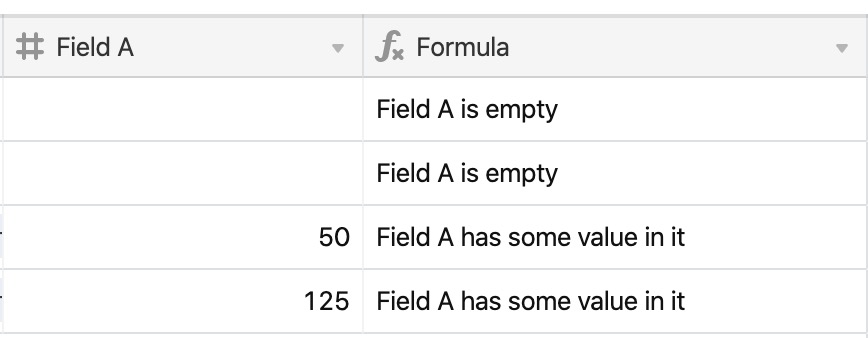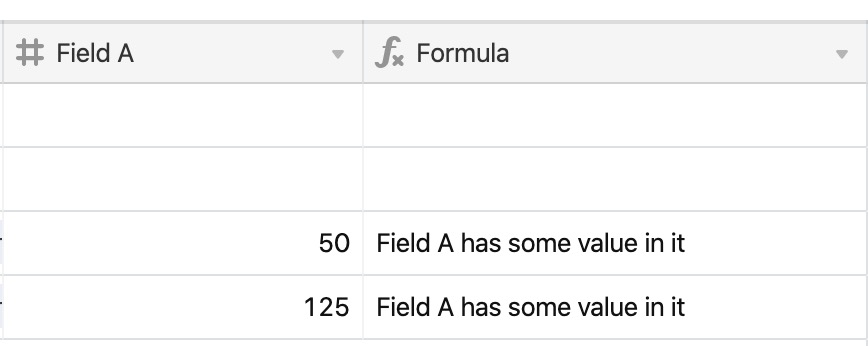• English
• Account
• API
• Integrations
• Enterprise
• Resources
Identifying blank values in Airtable
• 08 Nov 2023
• Dark
Light
• PDF

# Identifying blank values in Airtable

• Dark
Light
• PDF

Article Summary

This content is part of a guided course that includes the `BLANK()`function. The BLANK() function can identify an empty value in a field or output an empty value in a formula. Typically, the BLANK() function is used within IF statements.

 Plan availability All plan types Platform(s) Web/Browser, Mac app, and Windows app Related reading The essentials of Airtable formulas

## Finding an empty field

NOTE
When using BLANK() along with != (not equal to), you may receive unexpected results if the fields you are selecting aren't numeric.

To check if a field is empty using `BLANK()`, use a formula structure like this:

``````IF ({Field A} = BLANK() , "Field A is empty" , "Field A has some value in it" )
``````

If there is any value in the field, it will be evaluated as true and, otherwise, false.## Outputting a blank value

There are two ways to output a blank value in a field. Let's adjust the previously used formula only to output a message if `Field A` has a value.

``IF ({Field A} = BLANK(),  BLANK(),  "Field A has some value in it" )``As an alternative to using BLANK(), re-write the previously used formula to use empty quotes (`""`) instead.

``````IF (Condition, Value if True , "" )
``````

This will result in the exact same output as using `BLANK()`.

## Blank values in IF statements

When using IF statements, there are often times when you want to output some value if the condition evaluates as positive, and otherwise, show nothing. As a reminder, here is the structure of every basic IF statement:

``````IF(
Condition,
Value if true,
Value if false
)``````

You can use `BLANK() `to do so, like this:

``````IF(
Condition,
Value if true,
BLANK()
)``````

However, you can simplify that statement by removing the `ELSE `condition altogether:

``````IF(
Condition,
Value if true,
)``````